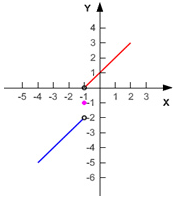Graphing
Example 2

The graph to the right is a graph of:
 {x - 1, if x < -1 (in blue)y = {-1, if x = -1 (in pink) {x + 1, if x > -1 (in red)The open dot at x = -1 (red line) shows that for the function x +1, x cannot be -1. At y = -1, there is just a dot because that is the only value x can be. For the function x - 1 there is an open dot because only the values where x is less than -1 are allowed.